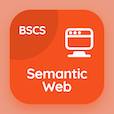Class 7 Online Courses

Grade 7 Science MCQs PDF - Topics

# Electrical Resistance MCQ Quiz Online

Practice Electrical resistance Multiple Choice Questions (MCQ) to solve electrical resistance quiz answers PDF to learn 7th grade science online course. Electric Circuits Multiple Choice Questions and Answers (MCQs), Electrical Resistance quiz questions PDF for online classes. "Electrical Resistance MCQ" PDF Book: electrical safety, electric current and units, electrical circuits test prep for online education.

"A component which provides resistance is called" Multiple Choice Questions (MCQ) on electrical resistance with choices heat, energy, product, and resistor for online classes. Learn electric circuits quiz questions for online certificate programs for virtual online school.

## MCQs on Electrical Resistance

MCQ: A component which provides resistance is called

heat
energy
product
resistor

MCQ: To control electric current in a circuit, there is used a

resistor
insulator
conductor
wire

MCQ: If more resistors are added in a series circuit the resistance will be

increased
decreased
equal
constant

MCQ: The resistor of a variable resistor can be changed hence it is called

ammeter
rheostat
fixed resistor
variable resistor

MCQ: In a parallel circuit the resistance decreases with increase in number of

resistors
bulbs
circuit
battery

### More Topics from Grade 7 Science Course